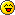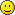# Determining Textures##Determining Textures

Hello againI found it would be better/more useful to others to ask this hear, rather than message you separately.
A little while back, when we were doing some comparisons of memory usage between Blockworld and my engine, you pointed out some obvious downfalls of mine. I now understand all of what you explained, except for one thing; you said: "I calculate texture coords inside my vertex shader based on the world coordinates and the direction the face is pointing". I was hoping you would be able to elaborate more on this?
Also, you mentioned sharing vertices between faces. My question here would be: How is this done if you store a single texture ID per vertex? If you were to have two separate Block Types right beside each other, would they or would they not share vertices? Thank you a ton in advance!##Re: Determining Textures

Hi there,

Assuming the 3d axis are laid out as follows; Y is vertical, X and Z are horizontal. Also
assuming the blocks vertices fall on integer values.

If the quad is facing upwards or downwards then the texture uses
the XZ coordinates. If the quad is facing north or south then the texture uses
the XY coordinates, and a quad facing east or west uses ZY as its texture
coordinates. I use a draw call for each direction of quad and I pass in the
direction (0..5). This is used to specify which pair of world coordinates to
use for texture coordinates.

I use Texture Arrays so each vertex only needs a ‘page
number’ in the texture array to say which texture to use.

If I have two different textures beside each other then I can’t
share those vertices, but I can if they are the same texture. I use a lattice
array to keep track of what vertices I have already generated and all their
info, and then when I am creating a new vertex I first check in the array to
see if I already have a matching vertex. If I do then I only get its index, I don’t
need to store another actual vertex.

##Re: Determining Textures

I did some tests my with my engine on the possibility to use the geometry shader.
What I did : Passing the faces as a "Points list", expanding the point into quad in the shader and computing the texture mapping based on the face type.

The result was : It drops clearly the qt of informations being send to the shader (Point list without text coord), but it was also 20% slower than with traditional way of doing (triangle lists with coord).

Did you see some performance impact on using the geometry shader ?

##Re: Determining Textures

I did use geometry shaders at one point but felt they slowed things down and eventually ditched them when i moved to smooth lighting. I also felt that on lower end hardware they might end up being really slow.

I have been using indexed triangle lists with just the texture number now for quite a while now.

##Re: Determining Textures

Thanks for the response! I am starting to understand more about how it works! I was under the impression that uses 6 face lists per chunk was testing, and it ended badly, but supposedly not?

If you are using the 6 lists thing, then your draw calls should be quite a bit smaller, so would this make room for a larger depth per chunk? Perhaps back to 256? Or does the number of added draw calls outweigh this idea?

Thanks for your helpPs: I will most likely come back to this a little later on, so if another reply is the result of that, that is why##Re: Determining Textures

I've been using the 6 calls per chunk pretty much since i started.

The chunk size has impacts on both drawing and rebuilding the meshes. Too tall a chunk and the meshes are slow to build, too small a chunk and draw time slows since you have to draw several chunks vertically to get a reasonable height.

Also, if you are drawing multiple chunks vertically then you have to consider the effects of sunlight which can penetrate all the way down to the bottom of the world and thus require a complete stack rebuild in some cases.

##Re: Determining Textures

My bad, I forgot about the relighting/rebuilding.I may be forgetting some obvious factor or something, but I am still confused on how you would go about deciding TexCoords in the vertex shader. Lets see if I can explain my problem:

There is going to be four points for each face of the block; Bottom Left (BL), Top Left (TL), Bottom Right (BR), and Top Right (TR). The UV coordinates are generally going to be:
BL = (0.0, 0.0)
TR = (0.0, 1.0)
BR = (1.0, 0.0)
TR = (1.0, 1.0)
My question is, how do you determine which vertex is which point on the face (BL,BR,TL,or TR) to allow you to choose the proper UV coordinates?

I hope I explained that properlyAlso, I am assuming that by using a Texture Array, you are meaning a 3D Texture. Using OpenGL (Me) vs. using DirectX (you) is sometimes hard to compare, haha##Re: Determining Textures

I use wrap for the tex co-ords.

So, for a quad my tex co-ords may be something like...

BL = (7, 7)
TL = (7, 8 )
BR = (8, 7)
TR = (8, 8 )

Because of wrap, these co-ords are interpreted as...

BL = (0, 0)
TL = (0, 1)
BR = (1, 0)
TR = (1, 1)

Now, the quad directly to the right of this one may have tex co-ords like so...

BL = (8, 7)
TL = (8, 8 )
BR = (9, 7)
TR = (9, 8 )

The X (world co-ord) is moved over by one, thus the U co-ord is moved over by 1 also.

Again, because of wrap, the co-ords are interpreted as...

BL = (0, 0)
TL = (0, 1)
BR = (1, 0)
TR = (1, 1)

I'm not 100% sure why this works, but it does.

I almost would have thought that the co-ords would have had to be something like...

BL = (8, 7 )
TL = (8, 7.999)
BR = (8.999, 7 )
TR = (8.999, 7.999)

for this to work properly.

##Re: Determining Textures

Okay, so from what I understand, what texture is displayed, is the difference from 0 or 1? So for

BL = (7, 7)
TL = (7, 8 )
BR = (8, 7)
TR = (8, 8 )

you would be using the 7th texture in your Texture Array? I hope I got that right hahaIf this is the case, I still don't understand is how you can determine which vertex is going to be the BL, or the TR, etc.

Sorry for being such a noob with this stuff##Re: Determining Textures

Oops, i forgot about the texture array part of the question. Sorry.

The stuff i posted above is just about getting the UV coords. There is a 'texture page' passed in as part of the vertex data. This is a third coordinate and is used with the UV to get the 'page' of the texture array.

Texture arrays are similar to 3d textures with one major difference. When you use mip maps with a 3d texture it mip maps the texture in 3d. When you do it with a texture array the mip mapping is not carried out between pages, only on each individual page.

I found an OpenGL reference to it's counterpart...

http://www.opengl.org/wiki/Array_Texture

##Re: Determining Textures

Thank you so muchAlso, thank you for finding the OpenGL reference! I don't know how I didn't find that when googling :/

Yes, I wasn't sure, but I was a little worried about 'bleeding' through on the images when reading into 3D textures, but I passed it off as a 'Dont worry about it for now' sort of thingI got the same 'page number' idea from reading over the 3D texturesSo, the first part about getting the UV coords, you do that instead of just using (0,0)(1,0)(0,1)(1,1) so your areas that share vertices can show a 'seamless' texture in between them? Like as in the cracks in the rock areas seem to line up perfectly with each other; I was wondering how you did thatAgain, thanks a million! You have been a huge help so far##Re: Determining Textures